# Samacheer Kalvi Books: Tamilnadu State Board Text Books Solutions

## Samacheer Kalvi 9th Maths Chapter 8 Statistics Notes PDF Download: Tamil Nadu STD 9th Maths Chapter 8 Statistics NotesSamacheer Kalvi 9th Maths Chapter 8 Statistics Notes PDF Download: Tamil Nadu STD 9th Maths Chapter 8 Statistics Notes

We bring to you specially curated Samacheer Kalvi 9th Maths Chapter 8 Statistics Notes PDF which have been prepared by our subject experts after carefully following the trend of the exam in the last few years. The notes will not only serve for revision purposes, but also will have several cuts and easy methods to go about a difficult problem.

 Board Tamilnadu Board Study Material Notes Class Samacheer Kalvi 9th Maths Subject 9th Maths Chapter Chapter 8 Statistics Format PDF Provider Samacheer Kalvi Books

## How to Download Samacheer Kalvi 9th Maths Chapter 8 Statistics Notes PDFs?

2. Click on the Samacheer Kalvi 9th Maths Notes PDF.
3. Look for your preferred subject.
4. Now download the Samacheer Kalvi 9th Maths Chapter 8 Statistics notes PDF.

## Tamilnadu Samacheer Kalvi 9th Maths Solutions Chapter 8 Statistics Additional Questions

Exercise 8.1

Question 1.
The following data gives the number of residents in an area based on their age. Find the average age of the residents.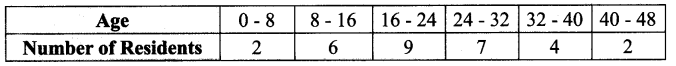Solution: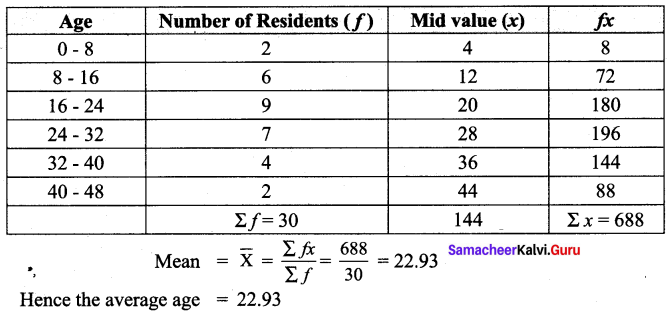Question 2.
Find the mean for the following frequency table :Solution:
Let Assumed mean A = 170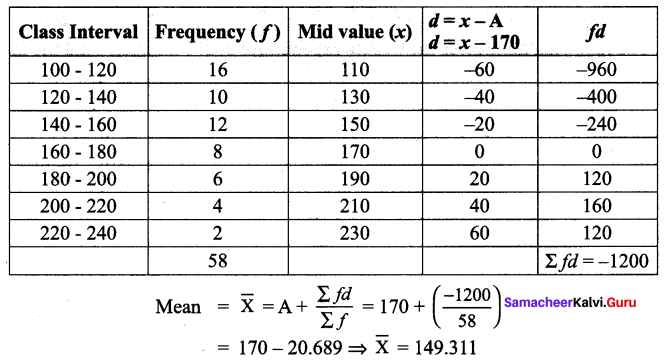Question 3.
Find the mean for the following distribution using step Deviation Method.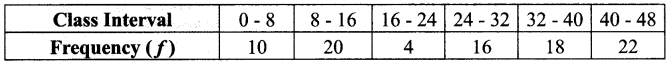Solution:
Let Assumed mean A = 28, Class width C = 8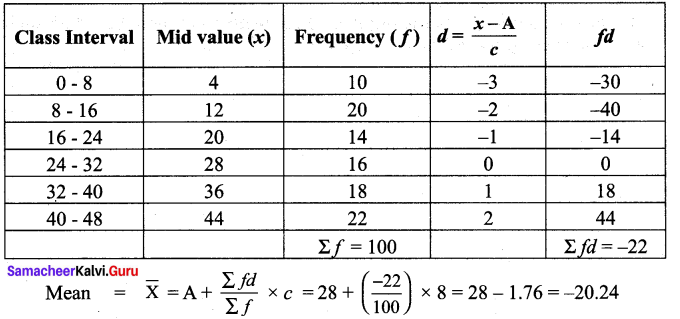Exercise 8.2

Question 1.
For the following up grouped data 8, 15, 14, 19, 11, 16, 10, 8, 17, 20. Find the median.
Solution:
Arrange the values in ascending order 8, 8, 10, 11, 14, 15, 16, 17, 19, 20
The number of values = 10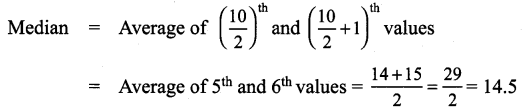Question 2.
The following table gives the weekly expenditure of 200 families. Find the median of the weekly expenditure.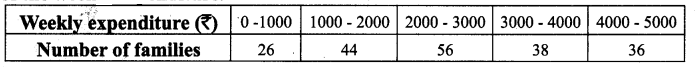Solution: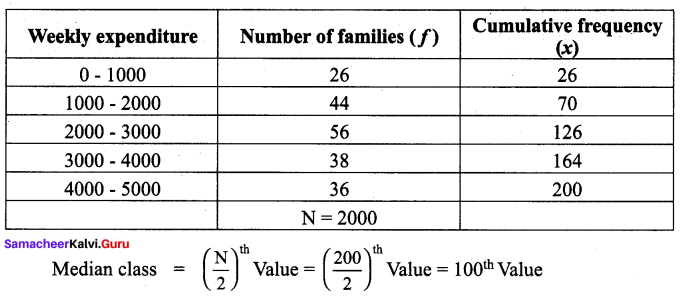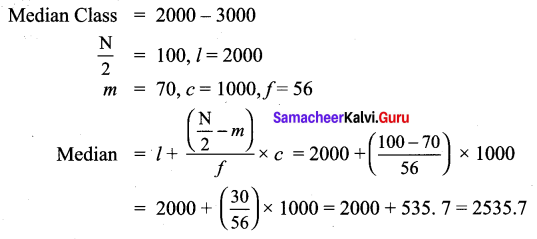Question 3.
The median of the following data is 24. Find the value of x.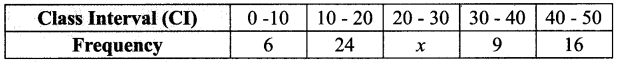Solution: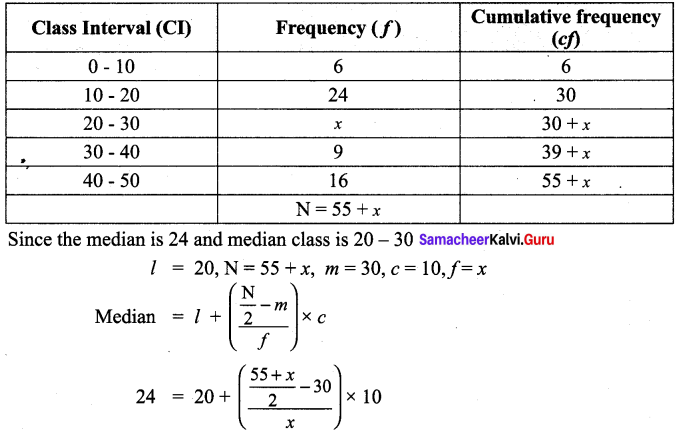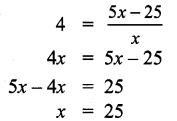Question 4.
The following are the scores obtained by 11 players in a cricket match 7, 21, 45, 12, 56, 35, 25, 0, 58, 66, 29. Find the median score.
Solution:
Let us arrange the values in ascending order 0, 7, 12, 21, 25, 29, 35, 45, 56, 58, 66
The number of values = 11 which is odd.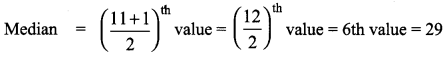Exercise 8.3

Question 1.
Find the mode of the given data : 65, 65, 71, 71, 72, 75, 82, 72, 47, 72.
Solution:
In the given data 72 occurs thrice. Hence the mode is 72.

Question 2.
Find the mode: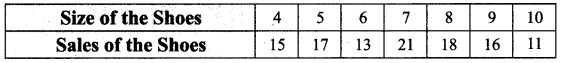Solution:
7 has the maximum frequency 21. Therefore 7 is the mode.

Question 3.
Find the mode for the following data.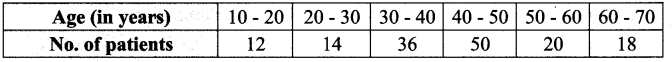Solution: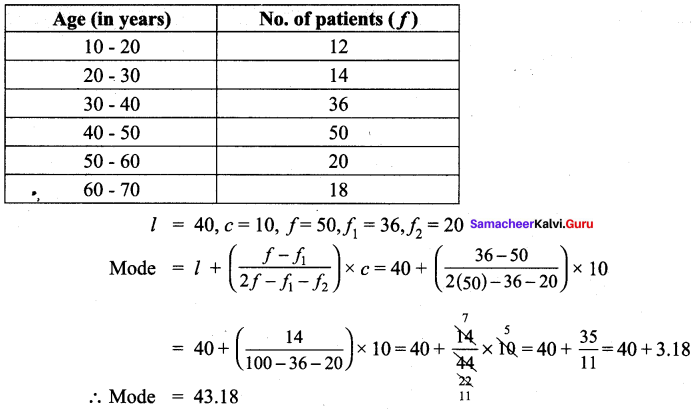Question 4.
In a distribution, the mean and mode are 46 and 40 respectively. Calculate the median.
Solution:
Given, Mean = 46 and mode = 40
Using mode ≈ 3 median – 2 mean ,
40 ≈ 3 Median – 2 (46)
3 Median ≈ 40 + 92
Therefore, Median ≈ 1323 = 44

Exercise 8.4

Multiple Choice Questions :

Question 1.
The mean of first 10 natural numbers.
(1) 25
(2) 55
(3) 5.5
(4) 2.5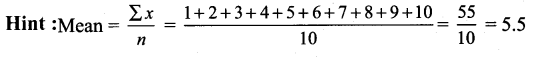Solution:
(3) 5.5

Question 2.
The mean of a distribution is 23, the median is 24 and the mode is 25.5. It is most likely that this distribution is :
(1) Positively skewed
(2) Symmetrical
(3) Asymptotic
(4) Negatively skewed
Hint: For Negatively skewed means is likely to be less than mode and median
Solution:
(4) Negatively skewed

Question 3.
The middle value of an ordered array of numbers is the
(1) Mode
(2) Mean
(3) Median
(4) Mid point
Solution:
(3) Median

Question 4.
The weights of students in a school is a :
(1) Discrete variable
(2) Continuous variable
(3) Qualitative variable
(4) None of these
Solution:
(2) Continuous variable

Question 5.
The first hand and unorganized form data is called
(1) Secondary data
(2) Organised data
(3) Primary data
(4) None of these
Solution:
(3) Primary data

## How to Prepare using Samacheer Kalvi 9th Maths Chapter 8 Statistics Notes PDF?

Students must prepare for the upcoming exams from Samacheer Kalvi 9th Maths Chapter 8 Statistics Notes PDF by following certain essential steps which are provided below.

• Use Samacheer Kalvi 9th Maths Chapter 8 Statistics notes by paying attention to facts and ideas.
• Pay attention to the important topics
• Refer TN Board books as well as the books recommended.
• Correctly follow the notes to reduce the number of questions being answered in the exam incorrectly
• Highlight and explain the concepts in details.

## Frequently Asked Questions on Samacheer Kalvi 9th Maths Chapter 8 Statistics Notes

#### How to use Samacheer Kalvi 9th Maths Chapter 8 Statistics Notes for preparation??

Read TN Board thoroughly, make separate notes for points you forget, formulae, reactions, diagrams. Highlight important points in the book itself and make use of the space provided in the margin to jot down other important points on the same topic from different sources.

#### How to make notes for Samacheer Kalvi 9th Maths Chapter 8 Statistics exam?

Read from hand-made notes prepared after understanding concepts, refrain from replicating from the textbook. Use highlighters for important points. Revise from these notes regularly and formulate your own tricks, shortcuts and mnemonics, mappings etc.
Share: